## research papersJOURNAL OFSYNCHROTRONRADIATION
ISSN: 1600-5775
Volume 14| Part 1| January 2007| Pages 169-172

## Verifying DiffEXAFS measurements with differential X-ray diffraction

aDepartment of Physics, University of Warwick, Coventry CV4 7AL, UK, and bEuropean Synchrotron Radiation Facility, 6 Rue Jules Horowitz, BP 220, 38043 Grenoble CEDEX, France
*Correspondence e-mail: m.p.ruffoni@warwick.ac.uk

(Received 3 August 2006; accepted 21 November 2006)

Differential EXAFS (DiffEXAFS) is a novel technique for measuring atomic perturbations on a local scale. Here a complementary technique for such studies is presented: differential X-ray diffraction (DiffXRD), which may be used to independently verify DiffEXAFS results whilst using exactly the same experimental apparatus and measurement technique. A test experiment has been conducted to show that DiffXRD can be used to successfully determine the thermal expansion coefficient of SrF2.

### 1. Introduction

Since the development of differential EXAFS (DiffEXAFS) (Pettifer et al., 2005), the study of small atomic perturbations has attracted much interest. Here we present a complementary technique for such studies: differential X-ray diffraction (DiffXRD).

This technique uses the same experimental apparatus and measurement technique as its EXAFS counterpart, such as that described by Pettifer et al. (2005) for magnetostriction measurements. The only difference, however, is that the sample through which transmission absorption measurements are taken (i.e. polycrystalline, amorphous etc.) is replaced by its single-crystal counterpart.

In kinematic diffraction theory, this introduces Laue diffraction features to the measured transmission intensity, where X-rays at certain energies are scattered out of the main line of the beam. These scattered photons do not enter the detector at the end of the beamline, causing an anomalous drop in transmitted intensity; seen as an apparent increase in X-ray absorption, which is observed as a discrete peak, independent of any true absorption fine-structure.

Such features make XAFS analysis difficult.1 However, being from diffraction in origin, they contain information on the structure of the sample material, and hence are sensitive to atomic perturbations in the same way as DiffEXAFS, albeit on a structurally averaged scale rather than a local atomic scale.

From Bragg's law and E = hc/λ, it is easy to show that, for a given diffraction peak,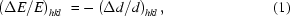where ΔE/E is the observed fractional change in peak position due to a relative change in interplanar spacing, Δd/d, corresponding to the Miller indices hkl. In the case of, for example, thermal expansion, this change is in turn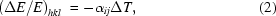where αij are the coefficients of the second-rank thermal expansion tensor, each of which can be obtained by analysis of an appropriate diffraction peak. In the case of cubic crystals, von Neumann's Principle dictates that properties such as thermal expansion are isotropic (Nye, 1985). The corresponding tensor therefore contains only one independent parameter; αij is reduced to α, and ΔE/E is the same for all diffraction peaks.

In order to accurately determine Δd using conventional X-ray diffraction techniques, it is typically necessary to vary the temperature of the sample by many tens of Kelvin between measurements such that a clear peak shift can be observed and thus measured. However, by utilizing the same measurement technique as DiffEXAFS (namely taking the difference between two spectra acquired in a short space of time under high-stability low-noise conditions, where the only change between measurements is the modulation of a given sample property), it is possible to detect extremely subtle shifts, and so obtain ΔE over temperature changes of the order of 1 K or less.

Thus, simply by substituting a DiffEXAFS sample with its single-crystal counterpart and repeating DiffEXAFS measurements, it is possible to obtain an independent measure of crystal perturbations without having to change any other part of the experimental set-up.

Interestingly, the resolution of DiffXRD features is limited by different factors than DiffEXAFS measurements. Pettifer et al. (2005) showed that DiffEXAFS is sensitive to atomic displacements of the order of femtometres, being limited by the achievable statistical noise in the spectrum. If the same noise were limiting a DiffXRD spectrum, it would theoretically be possible to detect signals from displacements of the order of 10−2 fm. However, such a feature would be produced from ΔE ≃ 10−7 eV, about two or three orders of magnitude smaller than the energy stability typically achievable between two absorption measurements taken a second or so apart. Thus, it is energy stability and energy resolution of the beamline that defines the smallest signal measurable by DiffXRD.

### 2. Experiment

DiffEXAFS experiments were conducted on ID24, the dispersive XAS beamline of the ESRF, as shown in Fig. 1(Pascarelli, Mathon et al., 2006).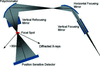Figure 1 Schematic representation of the optics on ID24 of the ESRF. A white beam from an undulator source illuminates a bent Si(111) polychromator crystal, creating a wavelength-dispersive fan of X-rays covering an equivalent energy range of several hundred eV. Diffraction by planes in the sample cause X-rays of certain wavelengths to scatter out of the main beam, which do not then enter the detector. This causes an apparent drop in transmitted X-ray intensity at those energies, and thus a diffraction glitch.

A single crystal of SrF2 (f.c.c. fluorite structure with a = 5.7996 Å) was cleaved along its 111 lattice planes, producing a section of size 5 mm × 5 mm and thickness 70 µm, and mounted at the focal spot of the beamline, producing an absorption jump of 1.9 at the Sr K-edge. This spectrum has been normalized to a unit jump and plotted in Fig. 2. Peaks generated by the effect of Laue diffraction removing flux from the beam are clearly present in the observed signal. Also plotted in Fig. 2is the DiffXRD signal, generated by subtracting the absorption signal shown from another taken with the sample heated by 1 K relative to it.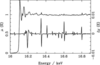Figure 2 The Sr K-edge measured in transmission through a single crystal of SrF2 (top plot, left-hand scale). The amplitude has been normalized to unit edge jump. Diffraction glitches are clearly present on the absorption fine-structure. As the temperature of the specimen is changed by 1 K at room temperature, these glitches shift in energy due to thermal expansion in the crystal, producing the DiffXRD signal shown (bottom plot, right-hand scale). Here, Δχ is the change in fine-structure amplitude, where χ is the normalized oscillatory component of μ above the edge by convention.

Such a change in sample temperature increases the size of the crystal unit cell according to thermal expansion. Given SrF2 has a cubic crystal structure, the thermal expansion is described by just one coefficient. The temperature shifts of all the diffraction peaks will, therefore, exhibit the same change in position independent of the Miller indices of the reflections. Indexing the diffraction features in Fig. 2is desirable, especially if dealing with a non-cubic crystal. Although achievable using a scheme detailed elsewhere (Pettifer & Hermes, 1985), this is a non-trivial task, requiring a goniometer to be attached to the sample that was not attached during this experiment.

The absence of any difference features at the same energy as the Sr K-edge in Fig. 2is testament to the energy stability of the beam between the two absorption measurements used to construct the DiffXRD signal. The observed differences are therefore from a genuine change in observed X-ray absorption rather than from drifts in the beam between measurements.

The diffraction peak at 16.35 keV, corresponding to the large symmetric feature in the DiffXRD signal, was extracted and transformed back to transmission space using I1/I0 = exp(−μx). A normalized Gaussian was then fitted to the transmission spectrum to determine its centroid energy, width at half-maximum and height. This fit is shown in Fig. 3, and the parameters are listed in Table 1. Other peaks showed an undesirable asymmetry of unknown origin.

 Table 1Fitted parameters for the diffraction peak shown in Fig. 3and for the corresponding DiffXRD feature shown in Fig. 4The thermal expansion coefficient has been derived using equation (2). Energies shown are not absolute energies, but based on a calibration with respect to another spectrum of known calibration. The errors shown are for the Gaussian and DiffXRD fits only and do not incorporate errors in calibration.
Parameter Value
Conventional Gaussian fit
Centroid (eV) 16349.505 ± 0.002
FWHM (eV) 2.137 ± 0.002
Relative height (−9.3782 ± 0.0003) × 10−2

DiffXRD fit
Centroid (eV) 16347.74 ± 0.07
Gaussian separation (eV) 1.84 ± 0.08
Thermal expansion (×10−6 K−1) 18.7 ± 0.8
†For a temperature modulation of 6.0 K.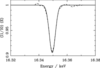Figure 3 The diffraction glitch (dashed line) at about 16.35 keV is extracted from the X-ray transmission spectrum, and the background subtracted. A Gaussian is fitted to the glitch (solid line) to determine its centroid energy, width at half-maximum and relative height.

It should be noted that, whilst diffraction peaks are Lorentzian in form, the energy resolution of ID24 at energies in excess of 15 keV causes some broadening, transforming them to Gaussians. Previous work has shown that an additional asymmetric component is also present (Ruffoni & Pettifer, 2006), caused by X-ray penetration-depth effects. However, we find that this does not make a significant contribution here.

Although the convolution of an instrument function with the diffraction signal clearly alters the peak's observed width and height and thus any fitted parameters, this is not a problem from the point of view of DiffXRD. As shown in equation (1), structural changes in the sample material are derived from a change in the measured energy of a given peak. Therefore, the only requirement for DiffXRD is that the peak retains its shape between the two measurements under different sample conditions, allowing this shift to be determined.

The DiffXRD signal, shown in Fig. 4, was obtained for a temperature change in the sample of 6 K rather than 1 K, increasing the degree of peak movement between measurements, and thus making the difference feature larger and easier to define. Given that the degree of movement is linearly related to the change in temperature by equation (2)(for small temperature changes), the energy shift per Kelvin may be restored by dividing the observed shift by the temperature difference. Using the Gaussian width and height parameters from Table 1to fix the shape of the diffraction peak to that seen in Fig. 3, the DiffXRD feature was characterized by calculating the difference in observed absorption between two such peaks, slightly offset in energy relative to one another, giving the separation shown at the bottom of Table 1. The centroid of the difference feature is defined as half way between the two constituent peak centroids, and thus is not the same as that of the initial Gaussian fit.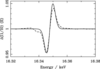Figure 4 The DiffXRD transmission signal obtained for ΔT = 6 K in the energy region of the glitch shown in Fig. 3(dashed line). The difference between a pair of Gaussians of width and height determined by the fit in Fig. 3, and offset in energy relative to one another, are fitted to the feature (solid line), the energy offset being related to the fractional change in lattice spacing.

Using equation (2), the thermal expansion coefficient of SrF2 was found to be (18.7 ± 0.8) × 10−6 K−1, in agreement with the published value of 18.1 × 10−6 K−1 at 300 K (Roberts & White, 1986).

### 3. Conclusions

It has been shown that DiffXRD is a viable technique for the study of average crystal perturbations, using an identical experimental arrangement as would be used for DiffEXAFS measurements.

Clearly, one advantage of DiffXRD over DiffEXAFS is the shear simplicity of the technique, equation (1)being considerably more straightforward to extract structural changes from than, for example, equation (2) from Pettifer et al. (2005) for DiffEXAFS.

Also, if the current trend of reducing focal spot sizes on beamlines continues (Pascarelli, Aquilanti et al., 2006), then in the future it will become easier to introduce diffraction phenomena into absorption spectra, making DiffXRD measurements simpler to perform. Additionally, if spot sizes decrease below about 1 µm × 1 µm, it will become possible to perform DiffXRD measurements on single crystallites in typical polycrystalline samples.

However, DiffXRD cannot be considered a replacement for DiffEXAFS in spite of this, since it is hindered by the same limitations that affect standard X-ray diffraction measurements. Firstly, the technique cannot be applied to disordered or amorphous systems with the same sensitivity to atomic positions as EXAFS, but, most importantly, structural information derived from DiffXRD is based on mean atomic perturbations over a periodic crystal structure. It does not yield information on local atomic perturbations, one of the real strengths of DiffEXAFS.

DiffXRD should therefore be considered complementary to DiffEXAFS. Simply by substituting a DiffEXAFS sample with its single-crystal counterpart (where available) and taking the same difference measurements with the same experimental apparatus, it is possible to obtain an independent measure of perturbations to the crystal structure to verify those from DiffEXAFS. Conversely, it could also be used to identify and quantify any discrepancies between local atomic perturbations and average (macroscopic) perturbations via a common experimental arrangement.

### Footnotes

1Diffraction features may be removed from XAS spectra using, for instance, the technique given by Tran et al. (2003).

### Acknowledgements

The authors would like to thank D. Sutherland, A. Lovejoy and A. Sheffield for their work in constructing apparatus for DiffEXAFS experiments, and S. Pasternak and F. Perrin for technical help whilst working on ID24.

### ReferencesJOURNAL OFSYNCHROTRONRADIATION